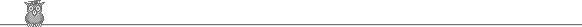### Putnam 1993Problem A4

Given a sequence of 19 positive (not necessarily distinct) integers not greater than 93, and a set of 93 positive (not necessarily distinct) integers not greater than 19. Show that we can find non-empty subsequences of the two sequences with equal sum.

Solution

Moderately hard. It is not clear how to proceed.

We establish the general case for 0 < x1 ≤ x2 ≤ ... ≤ xm ≤ n, 0 < y1 ≤ y2 ≤ ... ≤ yn ≤ m ≤ n.

Let ah = x1 + x2 + ... + xh, bk = y1 + y2 + ... + yk. Suppose that am ≤ bn (if not, we can just reverse the roles of x, a and y, b in what follows). For each i we have bn ≥ am ≥ ai, so we may define f(i) as the smallest j such that bj ≥ ai. Now consider the set {bf(1) - a1, bf(2) - a2, ... , bf(m) - am}. If any element is zero, then we are done, so we may assume that all elements are ≥ 1. Each element must be less than m, because if bf(i) ≥ ai + m, then bf(i)-1 >= ai, contradicting the minimality of f(i). So we have a set of m elements drawn from {1, 2, ... , m-1}. Hence there must be two elements, bf(r) - ar and bf(s) - as, which are equal. Assume r < s, then bf(s)-f(r) = as-r.